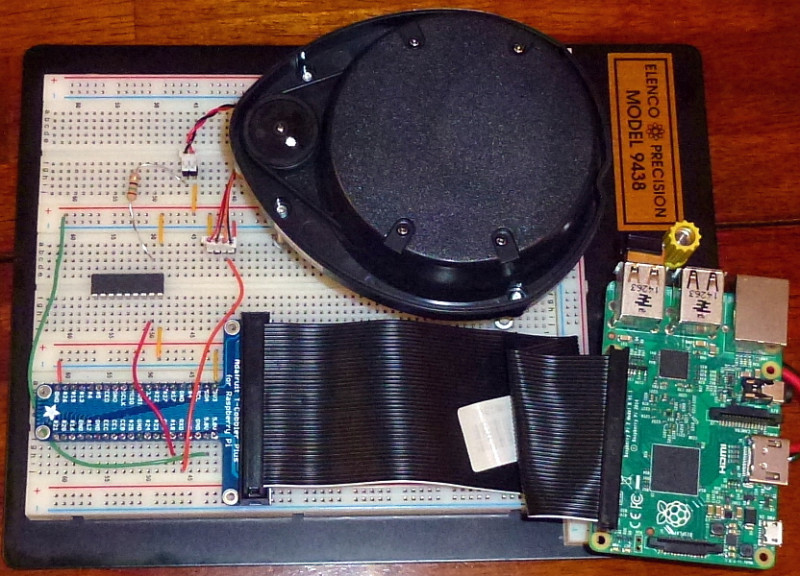# Tutorial: Raspberry Pi Lidar Visualization notesI’ve tried matplotlib which has a very convenient polar coordinate mode,

but the output looked very wrong, so there must be a few mistakes in here:

```#!/usr/bin/python
import matplotlib.pyplot as plt
import matplotlib.animation as animation
import serial
import numpy as np

com_port = "/dev/ttyACM0"
baudrate = 115200
ser = serial.Serial(com_port, baudrate)

fig = plt.figure(figsize=(4,4))
ax.set_ylim(0,2000)

data = np.zeros(360)
theta = np.linspace(0,360, num=360)
l,  = ax.plot([],[])

databuffer = ""

def update(i):
global data, databuffer
if (ser.inWaiting()>0):
databuffer +=  data_str
if ('A' in databuffer and 'B' in databuffer and 'C' in databuffer):
databuffer = databuffer.split('A')
databuffer = databuffer.split('C')
try:
(angle, distance) = databuffer.split('B')
ser.reset_input_buffer()
data[int(angle)]=int(distance)
except Exception as e: print(e)

l.set_data(theta, data )
return l,

ani = animation.FuncAnimation(fig, update, frames=360, interval=200, blit=True)
plt.show()```

#### Indian Software Outsourcing Giant Infosys Stunned Accounting Fraud Scandal, Stock Price Plummeted

This site uses Akismet to reduce spam. Learn how your comment data is processed.